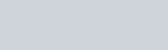1.4 for Android
App Name Geometry v1.4 Apps, Tools 5.0 MB 1.42020-11-05 1,000+

## Geometry

Ứng dụng Geometry nằm trong danh mục Tools

## Giới thiệu Geometry

Geometry Calculator is a powerful app which allows to calculate accurately the most important values and parameters of plane, 2D, 3D and solid figures and shapes.

It calculates all the missing parameters of all Geometry shapes including area occupied, height, all sides length, all missing angles ( acute, right, obtuse), radius (incircle and outcircle), Circumference, perimeter of shape, all diagonals and their length , Volume of solid shapes, arc length, base area, lateral surface area and total surface are of three dimensional geometric shapes using trigonometry, pythagorus theorem.

Simply type the values of known parameters, click calculate and the app will solve the Geometry shape for all parameters unknown values using trigonometric functions.

The Geometry calculator also shows the formulas and equations to simplify trigonometry learning easy.

List of shapes which can be solved are:-

Triangles
– scalene triangle
– Equilateral triangle
– right angle triangle
– isosceles triangle
– acute and obtuse triangle

– Square
– Rectangle
– Parallelogram
– Trapezium
– Rhombus
– Polygon

Curved 2d Shapes
– Circle
– Ellipse
– Annulus
– Torus

3D Shapes
– Parallel piped
– Cube
– Sphere
– Cylinder
– Cone
– Frustum of Cone
– Pyramid
– Frustum of pyramid
– Prism
– Octahedron

The Parameters and values which can be calculated are:-

Triangles:
– All sides including hypotenuse , perpendicular , base for all triangles )
– Area
– Perimeter
– Angles
– height
– Bisectors (for triangles )
– Medians (for triangles )
– Diagonals

– All unknown sides
– Angles
– Diagonals
– Diagonal Angles
– Area and perimeter
– Height

2d Curved Shapes:
– diameter
– arc length
– area
– circumference

3d Shapes
– volume
– Diagonals including volume diagonals
– Lateral surface area
– Base surface area
– total surface area
– height

Formulas for each parameter is also given. The formulas used are:
– Trigonometry
– Pythagorus theorem
and much more